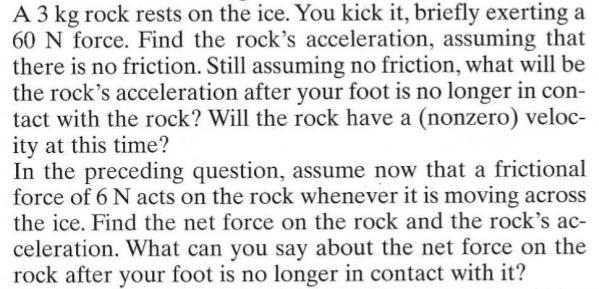Question:

# A 3 kg rock rests on the ice. You kick it, briefly exerting a 60 N force. Find the rock's acceleration, assuming that there is no friction. Still assuming no friction, what will be the rock's acceleraA 3 kg rock rests on the ice. You kick it, briefly exerting a 60 N force. Find the rock's acceleration, assuming that there is no friction. Still assuming no friction, what will be the rock's acceleration after your foot is no longer in contact with the rock? Will the rock have a (nonzero) velocity at this time? In the preceding question, assume now that a frictional force of 6N acts on the rock whenever it is moving across the ice. Find the net force on the rock and the rock's acceleration. What can you say about the net force on the rock after your foot is no longer in contact with it?